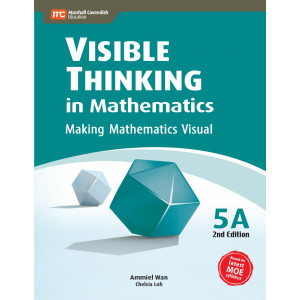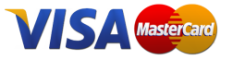﻿ Visible Thinking in Mathematics 5A - Singapore MathsView larger

IN STOCK

# Visible Thinking in Mathematics 5A

R151.32 VAT incl.

Visible Thinking in Mathematics - Making Mathematics Visual.   This is a series of supplementary books that promotes critical and creative thinking in mathematics. It is designed to help make thinking visible by providing the child with opportunities to think, explore and reflect.

### QuantityWhile practice leads to better performance, practice without contextual and conceptual understanding prevents the child from thinking critically and creatively. In this series, instead of learning procedures and formulas by rote, the child masters concepts through:

Thinking routines: functional questions to direct the child's thinking on key concepts and cultivate the child's thinking skills.

and

Parallel questions: consecutive mathematical problems with the same context but different key words to highlight differences between problems; ensuring that the child understands and retains concepts and skills better.

This approach not only instills in the child mathematical skills but also inspires discipline in thinking and greater motivation for learning. Additional support is provided to the child through Notes. Notes present opportunities for parents and teachers to clarify misconceptions, simplify difficult concepts and address areas of difficulty for the child.

Chapter 1
Numbers To 10 Million
Number Notation And Place Value
Reading And Writing Numbers In Words
Comparing And Ordering Numbers
Multiplication And Division By Tens, Hundreds And Thousands
Rounding Off And Estimation
Order Of Operations
Word Problems On Four Operations

Chapter 2
Fractions
Subtracting Unlike Fractions
Word Problems On Adding And Subtracting Unlike Fractions
Converting Fractions To Decimals
Subtracting Mixed Numbers
Word Problems On Adding And Subtracting Mixed Numbers
Multiplying Fractions
Dividing A Fraction By A Whole Number
Word Problems On Four Operations
Word Problems On Part-Whole Concept
Word Problems On Comparing Unlike Fractions

Chapter 3
Area Of A Triangle
Finding The Base And Height Of A Triangle
Finding The Area Of A Triangle
Finding The Area Of Triangles In Square Grids
Finding The Area Of Triangles In Composite Figures

Chapter 4
Ratio
Interpretation Of Ratio
Using Ratio In Measurement
Finding The Quantity Based On A Given Ratio
Equivalent Ratios
Word Problems On Equivalent Ratios
Finding The Ratio Of Three Quantities